# Design of Nonlinear Backstepping Control Strategy of PMSG for Hydropower Plant Power Generation

Design of Nonlinear Backstepping Control Strategy of PMSG for Hydropower Plant Power Generation

Abdelkader BoudaliKarim Negadi Sarah Bouradi Abderrahmane Berkani Fabrizio Marignetti

Laboratory of L2GEGI, Department of Electrical Engineering, University of Tiaret, BP 78 Zaaroura, Tiaret 14000, Algeria

Department of Automation, University Degli Studi of Cassino, Via G.Di Biasio 43, Cassino (FR) 03043, Cassino, Italy

Corresponding Author Email:
abdelkader.boudali@univ-tiaret.dz
Page:
1-8
|
DOI:
https://doi.org/10.18280/jesa.540101
Received:
6 July 2020
|
Accepted:
21 December 2020
|
Published:
28 February 2021
| Citation

OPEN ACCESS

Abstract:

In this paper, renewable hydropower plant generators with permanent magnet synchronous generator are coupled via a diode bridge rectifier - DC/DC boost converter and three-phase inverter to a power grid. This paper studies a new control structure focused a backstepping control of the energy generation system.The proposed methods for adjusting the active and reactive power by adjusting the currents, the DC bus voltage on the main side converter, as well as the voltage at the output of the DC-DC boost converter. The main objective of this control is to obtain purely sinusoidal and symmetrical grid current signals, to suppress oscillations in reactive power and to cancel active power chattering in the event of grid imbalance. In order to optimize the energy flow in the different parts of the production process, an energy control algorithm is developed in order to attenuate the fluctuations in the water flow, the grid system of the hydropower plant considered has been implemented in Matlab/Simulink, the results show the effectiveness of the proposed method. To analyze our approach, a prototype is modeled, simulated and can be performed in an experimental test setup.

Keywords:

PMSG, optimization, electrical grid, DC-DC boost converter

1. Introduction

Over the last years, the use of renewable energy has increased linearly, in an effort to avoid the pollution caused by the emission of green house gases, and also to face the exhaustion of the global reserves of fuels. Hence, the exploitation of more sustainable energy sources has become a hot topic in the last decade; in the form of hydroelectric systems, wind power systems, photovoltaic units, marine power systems, fuel cells, and biomass (etc.) .

Hydroelectric power plants (HPPs) are known to be a worldwide energy production unit; they cover around 20% of the total need of electricity in the world [2, 3].

One of the major drawbacks of the hydroelectric power system is its complexity containing hydraulic, mechanical, electrical and magnetic subsystems.The performance of power generation plants in terms of frequency control is of growing importance. Thus, the research on control strategies and dynamic processes of HPPs is of a major concern .

The hydraulic turbine is one of the basic constituents of the HPP conversion system; it lies mainly on the blades and the electric generator. The latter is responsible for converting the mechanical energy transmitted from the blades. The permanent magnet synchronous generators (PMSGs) are very often used in the hydroelectric power generation due to its high air-gap flux density, high power density, high torque to inertia ratio, and high efficiency . However, it is a nonlinear system and demands robust control; insensitivity to parameters variations or any external disturbances.

The backstepping control is an effective method for non-linear systems. It is based on the representation of the looped systems in the form of Lyapunov’s first order subsystems. In this context, the main interest of this control approach is to insert a control system to mitigate the disturbances due to water hammer in order to control the power of the hydraulic turbine and thus limiting it to its nominal value when the water flow is too high, to ensure maximum power extraction even at low water turbine speed, to regulate the reactive and active power independently, to sustain the frequency and voltage at the grid connection point within the qualified ranges, and to ensure the system operation at a unit power factor .

This paper is structured as follow: The description of the proposed system is discussed is Section 2. The Section 3 is dedicated to the hydroelectric turbine and its MPPT control. Section 4 deals with the advanced modelling of the electrical part. Section 5 discusses the backstepping controller. The simulation results ofthe study are shown in Section 6. Section 7 summarizes thework done in the conclusion.

2. Description of the Proposed System

The HPP is a three-part system containing a power plant where the electricity is produced; a dam that can be opened or closed in order to control the water flow; and a reservoir where the water is stored. The water behind the dam flows through an intake, and pushes against the blades of a turbine, causing them to rotate. The hydraulic turbine drives a synchronous generator to produce electricity. The amount of the electricity that can be generated depending on the extent to which the water drops and the amount of water circulating in the system. The energy produced feeds loads that can be composed of three or/and single-phase loads.

A small-scale hydropower station is usually a run-of-river plant that uses a fixed speed drive with mechanical regulation of the turbine water flow rate for controlling the active power generation. This design enables to reach high efficiency over a wide range of water flows but using a complex operating mechanism, which is in consequence expensive and tend to be more affordable for large systems. This paper proposes an advanced structure of a micro-hydropower plant (MHPP) based on a smaller, lighter, more robust and more efficient higher-speed turbine. The suggested design is much simpler and eliminates all mechanical adjustments through a novel electronic power conditioning system for connection to the electric grid. In this way, it allows obtaining higher reliability and lower cost of the power plant. A full detailed model of the MHPP is derived and a new three-level control scheme is designed. The dynamic performance of the proposed MHPP is validated through digital simulations and employing a small-scale experimental set-up. Figure 1 shows the various components of the constituent hydroelectric power plants .

## 1.jpg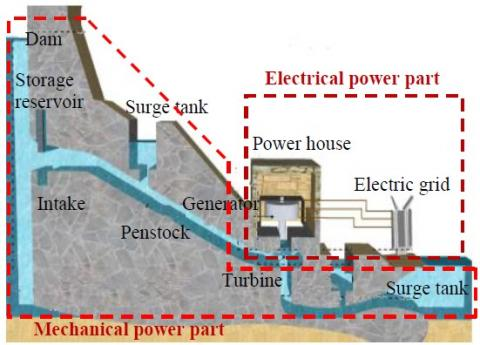Figure 1. Hydroelectric power generation components

3. Model of the Hydroelectric Turbine and Its MPPT Control

3.1 Model of the hydroelectric turbine

The power absorbed from the hydraulic turbine depends on the net water head H[m] and the water flow rate $Q_{\omega}\left[\mathrm{m}^{3} / \mathrm{s}\right]$ :

$p_{h}=\rho a H Q_{\omega}$  (1)

Hydraulic turbine efficiency $\eta$ is defined as the ratio of mechanical power transmitted by the shaft to the absorbed hydraulic power, which strongly affects the net output mechanical power $P_{m}[$ Watt $]$:

$p_{m}=\eta \rho a H Q_{\omega}$  (2)

where, $\rho\left[\mathrm{kg} / \mathrm{m}^{3}\right]$ is the volume density of water, g the acceleration due to gravity $\left[m / s^{2}\right]$.

The mechanical torque could be given by:

$T_{m}=\frac{P_{m}}{\omega}$  (3)

where, $\omega[\mathrm{rad} / \mathrm{s}]$ represents the turbine rotation speed.

According to equations of turbine model, the plot of the hydraulic turbine characteristics is shown in Figure 2. This plot is in function according to several values of mechanical and speed turbine.

3.2 MPPT control

The objective of this control technique is to guarantee the safety of the system and also to improve its efficiency, by maximising the power produced by the water flow; if it is below the reference value, otherwise; limiting the electrical power if it is above its reference . A large number of Maximum Tracking Power Points (MPPTs) methods and variety of converter topologies have been suggested in order to extract maximum power, namely Hill Climbing (HC), Perturb and Observe (P&O), Incremental Conductance (lnc Cond), and artificial intelligence techniques such as Fuzzy Logic and Neural Network .

The MPPT P&O algorithm is mainly used because of its ease of use, implementation and its low cost.

It is based on the following paradigm: as Figure 2 shows, if the operating voltage of the generator is disturbed in a given direction and $\frac{d P}{d \omega}>0$, it is known that the perturbation has moved the operating point to MPP, the P&O algorithm would then continue to disrupt the generator voltage in the same direction. Otherwise, if $\frac{d P}{d \omega}<0$, then the variation in operating point has moved away from the MPP, and the P&O algorithm reverses the direction of the disturbance.

## 2.jpg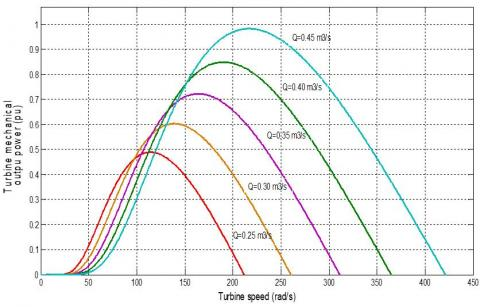Figure 2. Power-speed characteristics of a hydroelectric turbine

## 3.jpgFigure 3. MPPT power - speed characteristics

In other words that the system works by increasing or decreasing the operating voltage and observation of its impact on production power. The Perturb and Observe (P&O) system was implemented to extract the maximum power at all times. The voltage is disturbed at each MPPT cycle. As soon as the MPP is reached, it oscillates around the ideal of operation speed. For example, if the controller detects that the input the power increases $d P>0$ and the speed $d \omega>0$, it decreases the speed reference to bring it closer to the MPP [10, 11]. The Figure 3 explains the principle of the MPPT for a hydroelectric turbine with the power-speed characteristics.

3.3 DC-DC boost converters

The dc-dc converter acts as an interface between the rectifier and the network. A step-up converter is implemented here; it extracts the maximum power point whatever the variation in the water flow rate of the hydraulic turbine and the constraints applied to the electrical network. When changing the converter duty cycle, the source impedance can be matched to the load impedance to maximize power efficiency . This converter dampens or increases the output voltage relative to the input voltage.

4. Advanced Modeling of the Electrical Part

4.1 Permanent magnet synchronous generator model

The electrical model of the PMSG in the d-q frame linked to the stator rotating field is given by the system :

$\left\{\begin{array}{l}v_{s d}=R_{s} i_{s d}+\frac{d \psi_{s d}}{d t}-\omega_{r} i_{s q} \\ v_{s q}=R_{s} i_{s q}+L \frac{d \psi_{s q}}{d t}+\omega_{s} i_{s d}\end{array}\right.$  (4)

where,

$\left\{\begin{array}{l}\psi_{s d}=L_{d} i_{s d}+\psi_{s q} \\ \psi_{s q}=L_{q} i_{s q}\end{array}\right.$  (5)

The electromagnetic torque is given by:

$T_{e m}=p \cdot\left(\psi_{s d} \cdot i_{d}-\psi_{s q} \cdot i_{q}\right)$  (6)

4.2 Power converter

Power converter configuration for PMSG with diode rectifier three-phase and 2L-VSC is shown in Figure 4. This topology is cost-effective and provides a lightweight. The alternating output voltage of the generator is converted to direct current by the diode rectifier, the direct voltage is then reconverted to alternating voltage by 2L-VSC. The inductance of the intermediate circuit helps to smooth the output current of the diode rectifier. The output voltage of the hydraulic turbine and the diode rectifier changes with varying water flow conditions. However, the maximum value of the output voltage of the diode rectifier is limited by the nominal speed of the generator. Thus, the first dc link is referred to as the unregulated dc link. The output voltage of the diode rectifier becomes significantly weak at low water flow speed. The intermediate circuit voltage must be higher than the peak value of the line-to-line voltage of the grid to transfer the generated power to the grid. To ensure this condition, the generator must be overestimated (i.e. the generator nominal voltage must be higher than the that of the grid).

## 4.jpg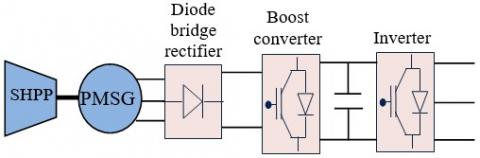Figure 4. Block diagram of the proposed hydropower plant energy conversion system

4.3 The grid model

Three-phase grid voltages are represented by the following equations :

$\left\{\begin{array}{l}v_{g a}=v_{\max } \cos (\omega t) \\ v_{g b}=v_{\max } \cos \left(\omega t-\frac{2 \pi}{3}\right) \\ v_{g c}=v_{\max } \cos \left(\omega t-\frac{2 \pi}{3}\right)\end{array}\right.$   (7)

The voltages in the d-q frame of reference are represented by the following equations:

$\left\{\begin{array}{l}v_{g d}=v_{i d}-R_{g} i_{g d}-L_{g} \frac{d i_{g d}}{d t}+\omega_{g} L_{g} i_{g q} \\ v_{g q}=v_{i q}-R_{g} i_{g q}-L_{g} \frac{d i_{g q}}{d t}-\omega_{g} L_{g} i_{g d}\end{array}\right.$  (8)

The power equations in the synchronous reference frame are given by :

$\left\{\begin{array}{l}P_{g}=\frac{3}{2}\left(v_{d g} i_{d g}+v_{q g} i_{q g}\right) \\ Q_{g}=\frac{3}{2}\left(v_{d g} i_{d g}-v_{q g} i_{q g}\right)\end{array}\right.$  (9)

The active and reactive power can be obtained after orienting the reference frame along the grid voltage from the following equations :

$\left\{\begin{array}{l}P_{g}=\frac{3}{2} v_{d g} i_{d g} \\ Q_{g}=\frac{3}{2} v_{d g} i_{q g}\end{array}\right.$  (10)

5. Design of the Backstepping Controller

The backstepping control is based on a multistep method, and at each stage, a virtual command is generated to ensure that the system converges to its equilibrium state. The stabilization of each synthesis step is ensured by the Lyapunov functions.

The synchronization of current with the mains voltages and the dc bus voltage adjustment are provided by the mains side converter, the backstepping control is used to control the active and reactive power supplied to the mains [15-18].

The grid currents in the d-q frame of reference can be expressed as follows:

$\left\{\begin{array}{l}\frac{d i_{g d}}{d t}=\frac{v_{i d}}{L_{g}}-\frac{R_{g}}{L_{g}} i_{g d}+\omega_{g} i_{g q}-\frac{v_{g d}}{L_{g}} \\ \frac{d i_{g q}}{d t}=\frac{v_{i q}}{L_{g}}-\frac{R_{g}}{L_{g}} i_{g q}-\omega_{g} i_{g d}-\frac{v_{g q}}{L_{g}}\end{array}\right.$  (11)

The module of the total voltage and current of the grid is established by:

$\left|v_{g}\right|=\sqrt{\left(v_{g d}\right)^{2}+\left(v_{g q}\right)^{2}}$

$\left|i_{g}\right|=\sqrt{\left(i_{g d}\right)^{2}+\left(i_{g q}\right)^{2}}$   (12)

The active and reactive powers in the d-q reference frame are given by:

$P_{g}=\frac{3}{2}\left(v_{g d} i_{g d}\right)=\frac{3}{2}\left(\left|v_{g}\right| i_{g d}\right)$

$Q_{g}=\frac{3}{2}\left(-v_{g d} i_{g q}\right)=-\frac{3}{2}\left(\left|v_{g}\right| i_{g q}\right)$   (13)

The control strategy applied to the GSC is based on making:

$v_{g q}=0$

$v_{g d}=\mid v_{g}\mid$  (14)

The powers equations become:

$P_{g}=\frac{3}{2}\left(v_{g d} i_{g d}+v_{g q} i_{g q}\right)$

$Q_{g}=\frac{3}{2}\left(v_{g q} i_{g d}-v_{g d} i_{g q}\right)$  (15)

The active and reactive powers dynamic is directly related to the components of the current.

The errors of the direct and quadrature grid current are defined by the following expressions:

$\left\{\begin{array}{l}e_{g d}=i_{g d}^{*}-i_{g d} \\ e_{g q}=i_{g q}^{*}-i_{g q}\end{array}\right.$  (16)

The error dynamics will be calculated as follows:

$\left\{\begin{array}{l}\dot{e}_{g d}=-\dot{i}_{g d} \\ \dot{e}_{g q}=-\dot{i}_{g q}\end{array}\right.$  (17)

The Lyapunov function according to the computed errors of the grid currents is determined as follows:

$V=\frac{1}{2}\left(e_{g d}^{2}+e_{g q}^{2}\right)$  (18)

The Lyapunov function dynamics can be derived as follows:

\begin{aligned} \dot{V}=&-K_{g d} e_{g d}^{2}-K_{g q} e_{g q}^{2} \\ &+e_{g d}\left(\frac{v_{i d}}{L_{g}}-\frac{R_{g}}{L_{g}} i_{g d}+\omega_{g} i_{g q}-\frac{v_{g d}}{L_{g}}+K_{g d} e_{g d}\right) \\ &+e_{g q}\left(\frac{v_{i q}}{L_{g}}-\frac{R_{g}}{L_{g}} i_{g q}-\omega_{g} i_{g d}-\frac{v_{g q}}{L_{g}}+K_{g q} e_{g q}\right) \end{aligned}  (19)

To obtain stability through this Lyapunov function, a positive value must be selected for the gains $K_{g d}$ and $K_{g q}$, and the derivative function must be negative. Consequently, we have to choose the reference voltages as follows:

$\left\{\begin{array}{l}v_{i d}^{*}=R_{g} i_{g d}-\omega_{g} L_{g} i_{g q}-L_{g} K_{g d} e_{g d}+v_{g d} \\ v_{i q}^{*}=R_{g} i_{g q}+\omega_{g} L_{g} i_{g d}-L_{g} K_{g q} e_{g q}+v_{g q}\end{array}\right.$   (20)

Eq. (21) provide the direct and quadrature current of the references as expressed by the following relationships.

The $i_{g q}^{*}$ current can be obtained from the following relation:

$i_{g q}^{*}=-\frac{2 Q_{g}^{*}}{3 v_{g q}}$  (21)

If the reference reactive power is forced to be zero $Q_{g}^{*}=0$, the system will operate with a unity power factor.

The calculation of the direct reference current igd-ref is obtained through the dc bus voltage regulation. The dc link voltage is regulated at the reference value $v_{d c}^{*}$, and if the converter losses are neglected, the transferred active power will be defined as follows:

$P_{g}=\frac{3}{2}\left(v_{g d} i_{g d}\right)=v_{d c} i_{d c}$  (22)

Thus, the $i_{g d}^{*}$ current is obtained from Eq. (22):

$i_{g d}^{*}=\frac{2 P_{g}^{*}}{3 v_{g d}}$  (23)

## 5.jpg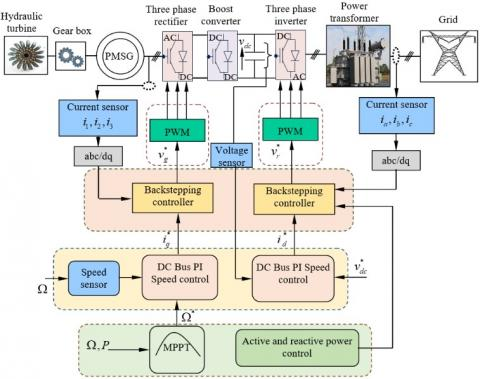Figure 5. Backstepping control scheme

The backstepping control structure for the GSC and the dc link voltage control is shown in Figure 5.

To control the dc bus voltage VC, a regulator must be implemented to maintain this voltage constant regardless of the current flow rate on the capacitor. The dc bus equation can be written as follows:

$P_{c}=c v_{d c} \frac{d v_{d c}}{d t}=P_{g}-P_{r}$  (24)

To control the dc voltage, we control the Pc in the capacitor by adjusting the power Pg using a conventional PI controller.

The block diagram of the simplified system to be controlled is presented in Figure 5.

6. Simulation Results and Discussion

As indicated in Figure 6, we propose in this study a water discharge flow rate varying between 0.75 m3/s and 1 m3/s. Simulations validation of the global control scheme were performed under the MATLAB/Simulink, in order to evaluate and test the proposed control technique for a complete model of hydropower plant based PMSG with power loop of current, active and reactive power and dc voltage using Backstepping controllers. The parameters are listed in Table 1 and 2 of the Appendix.

Hence, it is necessary to regulate the output parameters with the permissible limits. Therefore, it is proposed to implement ac-dc-ac converter which is of active front end type to improve the power quality of the system so that it can be connected to the grid.

Figure 7 shows the input rotation speed of the generator and its developed mechanical torque, as long as the torque is negative this means that, the machine operates in generator mode.

Figure 8 represents the output electromagnetic torque gives by the generator. In the Figure 9 it can be seen that the boost converter produces a fixed voltage 1200 V to power the inverter. The grid voltage and the dc link voltage are also found to be maintained under the same conditions of varying generator speeds as shown in Figures 10 and 11 respectively.

The grid voltages drop during water flow charging and it is improved to 600 V with the backstepping control strategy. The problem of voltage sagging can be eliminated with the help of a compensator.

## 6.jpg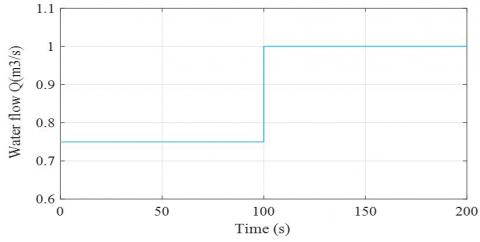Figure 6. Profile of variable flow rate

## 7.jpg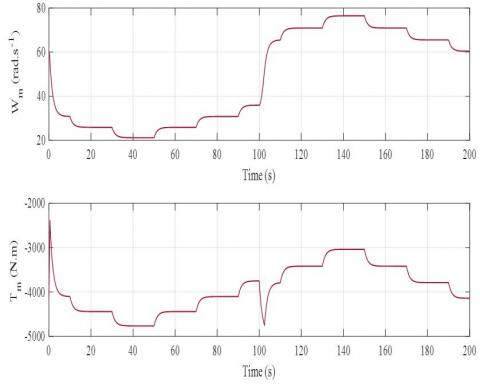Figure 7. The mechanical torque developed by the hydraulic turbine

## 8.jpg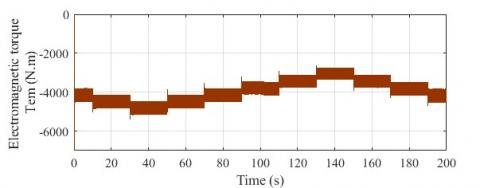Figure 8. Electromagnetic torque responses

## 9.jpg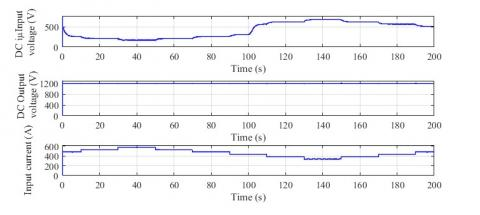Figure 9. The input/output current and voltage dynamics

## 10.jpg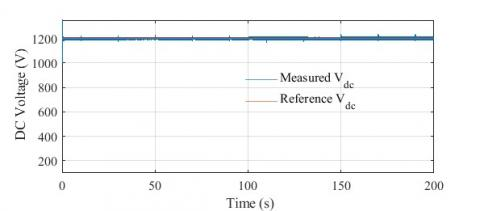Figure 10. The DC bus voltage

Due to the varying inputs, varying peaks are visible from the generator voltages. However, the grid voltage and the intermediate circuit voltage are kept constant.

The DC bus voltage perfectly follows its reference value as shown in Figure 10; this adjustment is ensured by the conventional PI regulator. Figure 12 shows the zoom of the grid voltage. They are purely sinusoidal and well balanced.

The Figures 11, 12, 13 and 14 show the current and the voltage of the grid with their zoom, they are purely sinusoidal which shows the efficiency of the control strategy applied to the converter which gives a better quality of energy to the grid.

From Figure 15, we note that the active and reactive powers follow their references with a transient regime very fast and without overtaking. As can be seen from Figure 15, the hydraulic turbine velocity is above the threshold, the power extracted is maintained in its rated values.

It can be seen that the current present variations of the power variations which shows that the current is the image of the powers.

## 11.jpg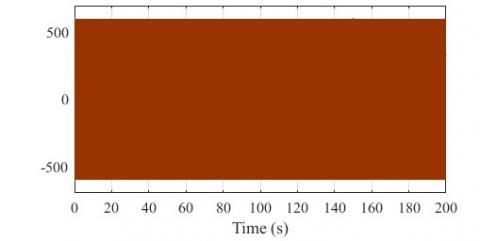Figure 11. The grid voltage

## 12.jpg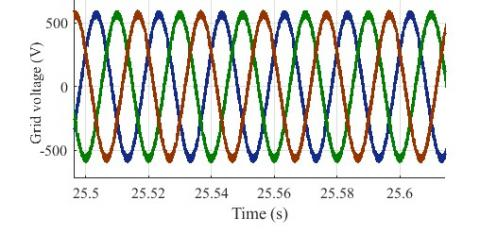Figure 12. Grid voltage zoom

## 13.jpg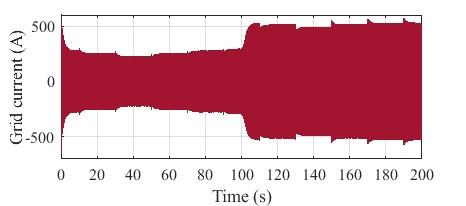Figure 13. The grid current responses

## 14.jpg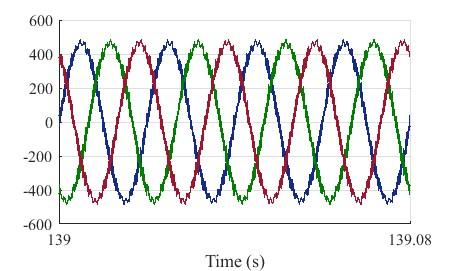Figure 14. Grid current zoom

## 15.jpg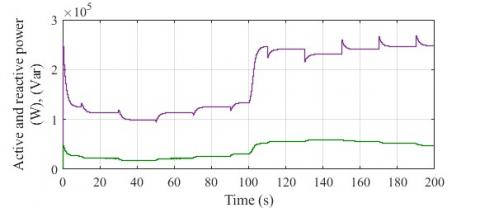Figure 15. Active and rective power

7. Conclusions

In this work a hydropower plant system connected to electrical grid via electronic power converters have been presented. Dynamic modeling, control and simulations of the proposed structure scheme have been done, using Matlab/Simulink. The energy system was developed and tested as well as its supervision-control setup. The combination of the hydraulic turbine and the PMSG, along with the diode bridge rectifier, DC-DC boost converter and a three phase inverter allow satisfying grid request. The conversion of the output of water flow structure into alternating current output is ensured by the use of a three phase three level inverter, these power converters are controlled to provide maximum output power under all operating conditions in an attempt to meet the grid requirements.

The backstepping control was also supported in this paper in an effort to maximize energy extraction from hydropower plant, to improve PMSG performance and to use the excess energy for internal loads and optimization of plant operation.

The results show the efficiency of the conversion chain and its control technique. The use of storage systems; namely the batteries and the super-capacitors; can be associated to the studied model in an eventual work. The proposed approaches can be implemented easily with DSP or Dspace platform.

Nomenclature
 ${{v}_{dg}},{{v}_{qg}}$ Grid peak voltages in dq-frame [V] ${{v}_{sd}},{{v}_{sq}}$ Thhe d, q components of the stator voltage ${{v}_{rd}},{{v}_{rd}}$ The d, q components of the rotor voltage ${{v}_{id}},{{v}_{iq}}$ Voltage inverter d, q components [V] ${{i}_{gd}},{{i}_{gq}}$ Grid peak currents in dq-frame [A] ${{i}_{sd}},{{i}_{sq}}$ The d, q components of the stator current ${{\psi }_{sd}},{{\psi }_{sq}}$ The d, q components of the stator flux ${{R}_{s}}$ The stator resistance ${{L}_{s}},{{L}_{r}}$ The stator and rotor inductance ${{\omega }_{s}},{{\omega }_{r}}$ The synchronous and rotor angular speed ${{\omega }_{sl}}={{\omega }_{s}}-{{\omega }_{r}}$ The slip angular speed ${{R}_{g}}$ Input filter resistance [Ω] ${{L}_{dg}},{{L}_{qg}}$ Input filter inductance d, q components [mH] ${{\omega }_{g}}$ Grid angular frequency [rad/s] ${{v}_{dc}}$ Dc bus voltage [V] $v_{dc}^{*}$ Reference Dc bus voltage [V] ${{i}_{dc}}$ DC bus current [A] $C$ DC link capacitor [mF] ${{R}_{s}}$ Load resistance [Ω] ${{P}_{g}}$ Grid active power [kW] $P_{g}^{*}$ Grid active power reference [kW] ${{P}_{c}}$ The power in capacitor ${{P}_{g}}$ Grid active power ${{P}_{h}}$ The power absorbed from the hydraulic turbine ${{P}_{m}}$ The mechanical power $p$ Number of pole pairs in a generator ${{Q}_{g}}$ Grid reactive power [kVAR] $Q_{g}^{*}$ Grid reactive power reference [kVAR] ${{Q}_{\omega }}$ Water flow [m3/s] ${{S}_{ai}}$ Switching state ${{T}_{em}}$ Electromagnetic torque [Nm] ${{T}_{s}}$ Sampling time [μs] $f$ Grid frequency [Hz] $H$ Water head [m] $\rho$ The volume density of water [kg/m3] $a$ The acceleration due to gravity [m/s2] $\omega$ The turbine rotation speed [rad/s] AC Alternatif Current DC Direct Current PMSG Permanent Magnet Synchronous Generator DSP Digital Signal Processor GSC Grid Side Converter HPP HydroPower Plant PWM Pulse Width Modulation VSC Voltage Source Converter MPPT Maximum power point tracking MPP Maximum power point

Appendix

Table 1. PMSG data used in simulation

 Components Rating values Rated power 250 kW Rated voltage Vrms 1,200 V Stator resistance 3 mΩ Armatur inductance 0.25 mH Inertia constant 0.1 e3kg.m2 Pole pairs 6 Rated frequency 50 Hz

Table 2. DC-DC converter parameters

 Components Rating values Rated voltage Vreference 1200 V Capacity rating 4.1 mF Inductance 5 mH
References

 Wei, L.J., Liu, Z.G., Zhao, Y.Y., Wang, G., Tao, Y.H. (2018). Modeling and control of a 600 kW closed hydraulic wind turbine with an energy storage system. Appl. Sci., 8(8): 1314. https://doi.org/10.3390/app8081314

 Guo, B.L., Seddik, B., Alamir, S., Mohamed, A. (2018). Variable speed micro-hydro power generation system: Review and experimental results. Symposium de Génie Electrique (SGE 2018), Nancy, France.

 Yang, W.J. (2017). Dynamic Processes and Active Power Control of Hydropower Plants, Thesis Uppsala University.

 Bouradi, S., Araria, R., Negadi, K., Marignetti, F. (2020). Nonlinear control of permanent magnet synchronous motor for high performances electric vehicle. Tecnica Italiana-Italian Journal of Engineering Science, 64(2-4): 317-324. https://doi.org/10.18280/ti-ijes.642-429

 Boudali, A., Negadi, K., Berkani, A., Boudiaf, M., Marignetti, F. (2020). Fuzzy logic control of DFIG small hydropower plant connected to the electrical grid. Tecnica Italiana-Italian Journal of Engineering Science, 64(2-4): 303-311. https://doi.org/10.18280/ti-ijes.642-427

 Guan, Y., Quintero, J.C.V., Guerrero, J.M., Wang, Y., Feng, W. (2015). Frequency stability of hierarchically controlled hybrid photovoltaic-battery-hydropower microgrids. IEEE Transactions on Industry Applications, 51(6): 4729-4742. https://doi.org/10.1109/TIA.2015.2458954

 Belhadji, L., Bacha, S., Roye, D. (2011). Modeling and control of variable-speed micro-hydropower plant based on Axial-flow turbine and permanent magnet synchronous generator (MHPP-PMSG). IECON 2011 - 37th Annual Conference of the IEEE Industrial Electronics Society, Melbourne, VIC, pp. 896-901. https://doi.org/10.1109/IECON.2011.6119429

 Zhou, W.J. (2017). Modeling, Control and Optimization of a Hydropower Plant. University College of Southeast Norway Faculty of Technology, Natural Sciences and Maritime Sciences, PhD dissertation in Process, Energy and Automation Engineering, Doctoral dissertation no. 33 2017.

 Ghoudelbourk, S., Dib, D., Omeiri, A., Azar, A.T. (2016). MPPT control in wind energy conversion systems and the application of fractional control (PIα) in pitch wind turbine. International Journal of Modelling, Identification and Control, 26(2): 140-151. https://doi.org/10.1504/IJMIC.2016.078329

 Lahfaoui, B., Zouggar, S., Elhafyani, M.L., Seddik, M. (2015). Experimental study of P&O MPPT control for wind PMSG turbine. 2015 3rd International Renewable and Sustainable Energy Conference (IRSEC), Marrakech, pp. 1-6. https://doi.org/10.1109/IRSEC.2015.7455020

 Soetedjo, A., Lomi, A., Mulayanto, W.P. (2011). Modeling of wind energy system with MPPT control. Proceedings of the 2011 International Conference on Electrical Engineering and Informatics, Bandung, pp. 1-6. https://doi.org/10.1109/ICEEI.2011.6021836

 Araria, R., Berkani, A., Negadi, K., Marignetti, F., Boudiaf, M. (2020). Performance analysis of DC-DC converter and DTC based fuzzy logic control for power management in electric vehicle application. Journal Européen des Systèmes Automatisés, 53(1): 1-9. https://doi.org/10.18280/jesa.530101

 Borkowski, D. (2018). Analytical model of small hydropower plant working at variable speed. IEEE Transactions on Energy Conversion, 33(4): 1886-1894. https://doi.org/10.1109/TEC.2018.2849573

 Krim, Y., Abbes, D., Krim, S., Mimouni, M.F. (2018). Classical vector, first-order sliding mode and high-order sliding-mode control for a grid-connected variable speed wind energy conversion system: A comparative study. Wind Engineering, 42(1) 16-37. https://doi.org/10.1177/0309524X17723202

 Youness, E.M., Aziz, D., Abdelaziz, E.G., Jamal, B., Najib, E.O., Othmane, Z., Khalid, M., Bossoufi, B. (2019). Implementation and validation of backstepping control for PMSG wind turbine using dSPACE controller board. Energy Reports, 5: 807-821. https://doi.org/10.1016/j.egyr.2019.06.015

 Oğuz, Y., Güney, İ., Çalık, H. (2013). Power quality control and design of power converter for variable-speed wind energy conversion system with permanent-magnet synchronous generator. The Scientific World Journal, 2013: 783010. https://doi.org/10.1155/2013/783010

 Doumi, M., Aissaoui, A.G., Tahour, A., Abid, M., Tahir, K. (2016). Nonlinear integral backstepping control of wind energy conversion system based on a Double-Fed Induction Generator. Przegląd Elektrotechniczny, 92(3): 130-135. https://doi.org/10.15199/48.2016.03.32

 Errami, Y., Obbadi, A., Sahnoun, S., Benhmida, M., Ouassaid, M., Maaroufi, M. (2016). Design of a nonlinear backstepping control strategy of grid interconnected wind power system based PMSG. In AIP Conference Proceedings, AIP Publishing LLC, 1758(1): 030053.